# AP Chemistry Practice Test 23

### Test Information12 questions18 minutes

1. Chemical reactions can be classified as either heterogeneous or homogeneous. Which of the following equations below is best classified as a heterogeneous reaction?

2. Which of the reactions below will be thermodynamically favored at all temperatures?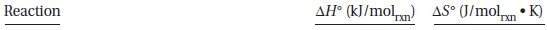3.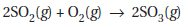Consider the following possible mechanism for the reaction above: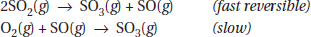Which of the following statements is true?

4.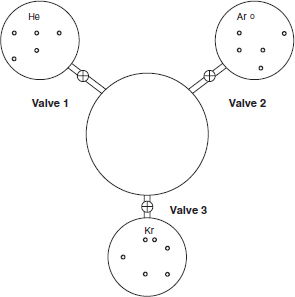Three 1-liter flasks are connected to a 3-liter flask by valves. The 3-liter flask is evacuated to start and the entire system is at 298K. The first flask contains helium, the second argon, and the third krypton. The pressure of the argon is 633 torr. The amounts of gas are proportional to their representations in the flasks. If all the valves to the center flask are opened, what will the pressure of the system be? Assume the connections have negligible volume.

5.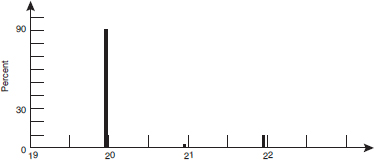A portion of a mass spectrum of neon is presented above. Estimate the average mass of naturally occurring atoms of neon, assuming that the height of each line represents the relative amount of each mass.

6. Which of the following particulate diagrams best represents the reaction of carbon monoxide with oxygen to form carbon dioxide?

`Questions 7-11 refer to the following information.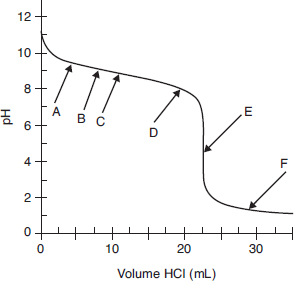The above graph shows a titration curve of a weak base titrated with a strong acid. The pH was measured with a pH meter after small volumes of 0.075 M HCl were added to 25.0 mL of a weak base. Data from that experiment are shown in the graph.`

7. Which arrow points to the end point of this titration?

8. Which arrow points to the place on the curve where the pH is equal to 14 - pKb?

9. If the student used a pH indicator that changes color from pH 5 to 7, which statement best characterizes the expected observations?

10. Find the two points on the curve that indicate the region where the solution can be described as a buffer. What is the change in pH from the first point to the second?

11. Which of the following describes the base that is being titrated?

12. Methylamine, CH3NH2, has a Kb = 4.4 × 10-4. If a 0.0100 M solution of methylamine is prepared, the expected pH will be in which one of the following pH ranges?